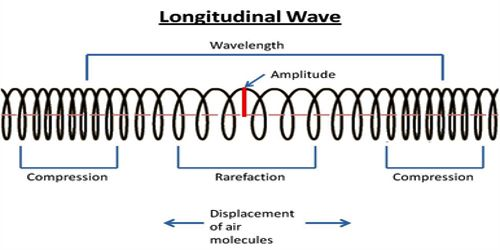# Characteristics of Longitudinal Wave

When particles of the medium vibrate about their mean position parallel to the direction of propagation of the disturbance, the wave is called a longitudinal wave. It is a wave where the particles in the medium move parallel to the direction of propagation of the wave. When particles of the medium vibrate about their mean position parallel to the direction of propagation of the disturbance, the wave is called a longitudinal wave. This waves are the particles of the medium move parallel to the wave’s direction of travel.

Examples: The various examples of a sound wave are: Sound wave, Waves in a slink, Glass vibrations, Ultrasound, Spring oscillations, Waves in spring, seismic P-waves, etc.

Characteristics of longitudinal wave

• The wave in which the particles of the inanimate medium vibrate about their mean position to the direction of propagation of the disturbance, the wave is called the longitudinal wave.
• During the flow of the wave compression and rarefaction of the medium take place.
• Distance between two successive compressions or contraction or combined distance of compression and rarefaction is called wavelength.
• Compression is a region in a longitudinal wave where the particles are closest together and rarefaction is a region in a longitudinal wave where the particles are furthest apart.
• The polarization of the medium does not take place.
• This wave generates an elastic medium.
• The period of a wave is the time taken by the wave to move one wavelength and the frequency of a wave is the number of wavelengths per second.
• In longitudinal waves, the displacement of the medium is parallel to the propagation of the wave.
• The wavelength in a longitudinal wave is the distance between two consecutive points that are in phase. The wavelength refers to the distance between two consecutive compressions or between two consecutive rarefactions.
• For longitudinal waves, the vibration of the particles of the medium is in the direction of wave propagation.
• The amplitude is the utmost disarticulation from a position of rest. It is the distance from the balance position of the intermediate to compression or rarefaction.
• As in the case of transverse waves the following properties can be defined for longitudinal waves: wavelength, amplitude, period, frequency and wave speed.
• Examples of these waves are sound waves, tsunami waves, earthquake P waves, ultrasounds, vibrations in gas, and oscillations in spring, internal water waves, and waves in slink, etc. these waves can move through air, solids or liquids.